# Standard enthalpy of sublimation

Chemical Thermodynamics: Standard enthalpy of sublimation

Sublimation is a process in which a solid material converts from its solid state to vapour state without passing through the liquid state. It is an endothermic reaction which occurs at pressures and temperature below a substance’s triple point. In thermodynamics, the triple point of a substance is a pressure and temperature point at which all three states/phases (solid, liquid and gas) can coexist in thermodynamic equilibrium. For instance, when naphthalene balls are in a solid dorm, their molecules have no intermolecular space. This means that there is no movement of particles and they have no kinetic energy. But, when these molecules gain energy, the molecules move randomly and their intermolecular space increases changing the solid balls to gaseous form. Scientists can measure the heat required for this conversion and then express the result as the heat of sublimation. It is usually denoted in units of joules of heat per gram of a substance, J/g, or joules per mole of a substance, J/mol. Also, note that sublimation is applicable only when a purely physical change of state in a substance takes place. Which means that any chemical reaction that results in the formation of gas is not necessarily a sublimation process. In this process, the latent heat of sublimation is corresponding to the reaction.Figure : Heat exchange and change of state in particles.

Enthalpy of sublimation is the change in enthalpy of a substance when 1 mol of its solid dorm sublimes and converts to a gas at standard temperature and pressure (1 atm and 298 K). Sublimation requires all intermolecular forces between the molecules (or other species, such as ions) in the solid to be broken to convert it into a gas. The heat of sublimation is expressed as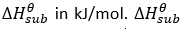is always greater than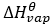since vaporization is the conversion of molecules of a substance from liquid state to a gaseous state. aFor example, the sublimation of CO2 is represented by: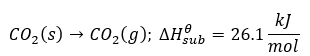Since enthalpy of fusion of any substance is less than it enthalpy of vaporization, we may consider sublimation as a two-step process of melting followed by evaporation. We know that enthalpy is a state function which means path taken by a substance for its transformation is not important when it changes from one state to another. Here we can apply Hess’s Law to obtain enthalpy of sublimation by equating the other two latent heats. Though not accurate, the estimation is very close to the real number and logically acceptable.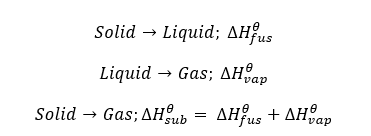Where enthalpy of fusion is the change in heat when 1 mole of a solid substance is converted to a liquid state at its melting point. Enthalpy of vaporization is the change in heat when 1 mole of a liquid substance is converted to a gaseous state at its boiling point.

For example,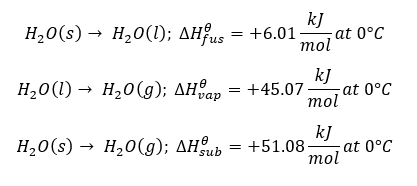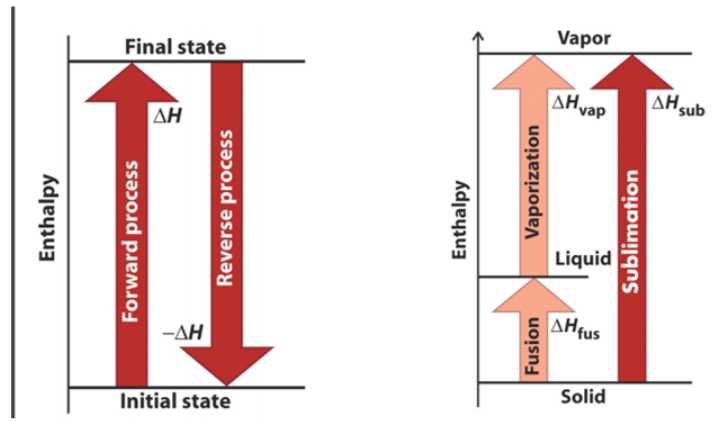Figure : Consequences of enthalpy being a state function. For a given substance, the sum of enthalpy of vaporization and fusion is approximately equivalent to the enthalpy of sublimation.